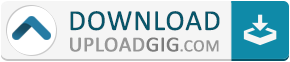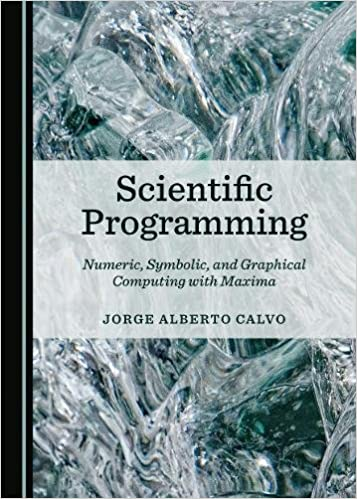#Do not remember me!

### Categories» » » Scientific Programming: Numeric, Symbolic, and Graphical Computing with Maxima

## Scientific Programming: Numeric, Symbolic, and Graphical Computing with MaximaEnglish | ISBN: 1527511170 | 2018 | 562 pages | PDF | 7 MB

This book offers an introduction to computer programming, numerical analysis, and other mathematical ideas that extend the basic topics learned in calculus. It illustrates how mathematicians and scientists write computer programs, covering the general building blocks of programming languages and a description of how these concepts fit together to allow computers to produce the results they do. Topics explored here include binary arithmetic, algorithms for rendering graphics, the smooth interpolation of discrete data, and the numerical approximation of non-elementary integrals. The book uses an open-source computer algebra system called Maxima. Using Maxima, first-time programmers can perform familiar tasks, such as graphing functions or solving equations, and learn the basic structures of programming before moving on to other popular programming languages. The epilogue provides some simple examples of how this process works in practice. The book will particularly appeal to students who have finished their calculus sequence.Name:* E-Mail: Security Code: *